Latest Banking jobs   »

# Quantitative Aptitude Quiz For IBPS RRB PO/Clerk Prelims 2023 -26th May

Directions (1-5): – In each of the following questions, two equations (I) and (II) are given, you have to solve both the equations and give answer.
(a) If x > y
(b) If x ≥ y
(c) If x < y
(d) If x ≤ y
(e) If x = y or no relation can be established betw6een x and y.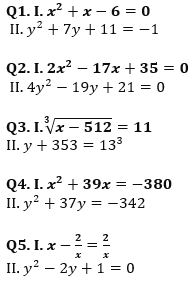Directions (6-10): In each of the following questions two equations (I) and (II) are given, you have to solve both the equation and give answer.
(a) if x < y
(b) if x ≤ y
(c) if x > y
(d) if x ≥ y
(e) if x = y or no relationship can be obtained between x and y.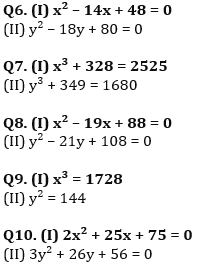Solutions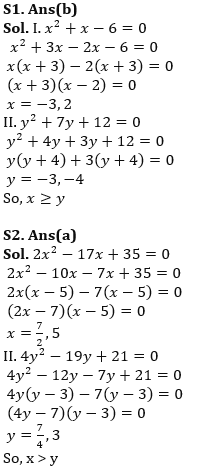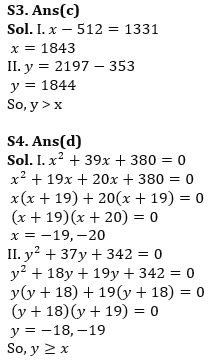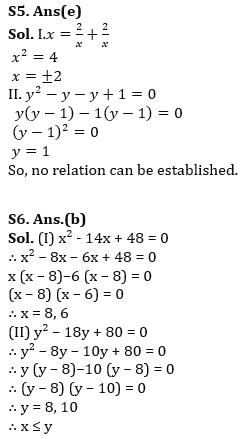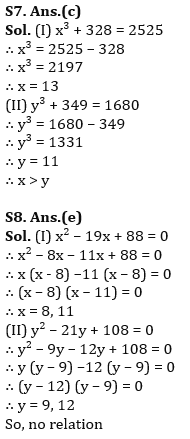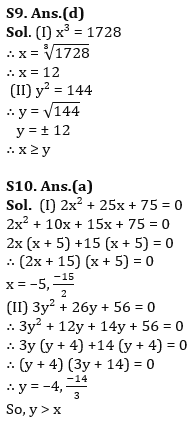.                                                                                                                                                                             .

## FAQs

### What is the selection process of IBPS RRP PO?

The Selection Process of IBPS RRB PO consists of Prelims, Mains and Interview

#### Congratulations!Union Budget 2023-24: Free PDF